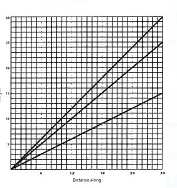# Lesson plan

Piagetian Level Learners Thinking and abstract of the activity
3 B
Mature
FormalUse of proportional reasoning

'we found that each slide had a constant ratio between height and length so the distances along the ground will give us how much higher the cathedral is than our stick'
3A
Early
Formal
Ratio as a relationship between numbers.
'multiply A by 3/2'
'each A is two-thirds of C'
'multiply A by 3 then halve it'
'Junior to Infant is 5 to 3 so you multiply by 5 and divide by 3'

'the Junior is 5/6ths as high as the Senior'

'for every 5 the Junior goes up the Senior goes up 6'
'I think if you made a scale diagram like our slides you could measure it'
2B*
Concrete
Generalisation
'halve A and add it to itself''divide B by 2 to get C: multiply C by 2 to get B'

'B goes up in 6's; C goes up in 3's'

'each C is half the B'
Mid
concrete
Episode 1
Multiple relationships

Episode 2
Playground slides
Episode 3
Height of cathedral